ICSE Class 9 Circle MCQ New

## ICSE Class 9 Circle MCQ New Pattern

ICSE Class 9 Circle MCQ By Clarify Knowledge

## ICSE Class 10 All Subject MCQ

### By solving the inequality 3(a - 6) < 4 + a, the answer will be

• A.a < 9
• B.a < 12
• C.a < 13
• D.a < 11

Solution:QUESTION: 2

### What is the solution set for• A.(−12,∞)
• B.(−44,∞)
• C.(−24,∞)
• D.(−4,∞)

Solution:QUESTION: 3

### Identify the solution set for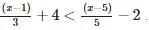• A.(−∞,−50)
• B.(−∞,−5)
• C.(−∞,-10)
• D.(−∞,−15)

Solution:QUESTION: 4

What is the solution set for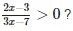• A.• B.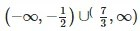• C.• D.Solution:QUESTION: 5

Identify the solution set for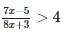• A.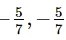• B.• C.• D.none of these.

Solution:

(7x-5)/(8x+3) > 4
(7x-5)/(8x+3) - 4 >0
7x - 5 - 4 ( 8x + 3 ) / 8x + 3 > 0
- 25 x - 17 / 8x + 3 > 0
Now furthermore solving for general range :
x ∈ ( -17/ 25, - 3/8)QUESTION: 6

What is the solution set for• A.(5 , 6)
• B.(- 6 , 6)
• C.(- 6 , 0)
• D.none of these

Solution:QUESTION: 7

Identify solution set for | 4 − x | + 1 < 3?

• A.(2 , 6)
• B.(3 , 6)
• C.(2 , 4)
• D.(2 , 3)

Solution:

|4 − x| + 1 < 3
⇒ 4 − x + 1 < 3
Add −4 and −1 on both sides, we get
4 − x + 1 − 4 − 1 < 3 − 4 − 1
⇒ − x < −2
Multiply both sides by −1, we get
x > 2
Also,|4−x| + 1 < 3
⇒ −(4−x) + 1 < 3
⇒ − 4 + x + 1 < 3
Add 4 and −1 on both sides, we get
− 4 + x + 1 + 4 − 1 < 3 + 4 − 1
⇒ x < 6
Thus, x ∈ (2,6).QUESTION: 8

What is the solution set for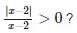• A.(2 , ∞)
• B.(0 , 2)
• C.(-∞ , - 2)
• D.none of these

Solution:

|x-2|/(x-2) > 0
=> x - 2 > 0
x > 2
x denotes (2,∞)QUESTION: 9

Identify the solution set for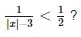• A.(−∞,−5) ∪ (5,∞)
• B.(5,∞)
• C.(−∞,−5) ∪ (−3,3) ∪ (5,∞)
• D.none of these

Solution:

Explanation:- 1/|x| - 3 < ½

By cross multiply, we get

2 > |x| - 3

2+3 <|x|

5 < |x|

(5,∞)QUESTION: 10

What is the solution set for• A.(- 3/4 ,1) ∪ (⁡1,∞)
• B.(3/4,1) ∪ (⁡1,∞)
• C.(1/4,1) ∪ (⁡1,∞)
• D.none of these

Solution:

|(2x-1)/(x-1)| > 2
|x| > a
⇒ x > a
or x < -a
(2x-1)/(x-1) > 2 and (2x-1)/(x-1) < -2
(2x-2+1)/(x-1) > 2
⇒ (2(x-1) + 1)/(x-1) > 2
⇒ 2 + (1/(x-1)) > 2
1/(x-1) > 0
x-1 < 0
x < 1...........(1)
Now taking, (2x-1)/(x-1) < -2
2 + (1/(x-1) < -2
= 1/(x-1) < -4
x-1 > -1/4
x > -1/4 + 1
x > 3/4.......(2)
From (1) and (2)
x implies (3/4, 1)∪ (⁡1,∞)QUESTION: 11

In the first four papers each of 100 marks, Rishi got 95, 72, 73, 83 marks. If he wants an average of greater than or equal to 75 marks and less than 80 marks, find the range of marks he should score in the fifth paper .

• A.52 ≤ × < 77
• B.73 < × < 100
• C.25 < × < 75
• D.75 < × < 80

Solution:

Let marks in 5th paper be x
Average = (95 + 72 + 73 + 53 + x)/5
= (323 + x)/5
(75 ≤ 323 + x)/5 < 80
375 ≤ 323 + x < 400
52 ≤ x < 77
[52,77).QUESTION: 12

A man wants to cut three lengths from a single piece of board of length 91cm. The second length is to be 3 cm longer than the shortest and third length is to be twice as long as the shortest. What are the possible lengths for the shortest board if the third piece is to be at least 5 cm longer than the second?

• A.5 ≤ × ≤ 91
• B.3 ≤ × ≤ 5
• C.3 ≤ × ≤ 91
• D.8 ≤ × ≤ 22

Solution:QUESTION: 13

Find all pairs of consecutive odd natural numbers, both of which are larger than 10, such that their sum is less than 40.

• A.(11 , 13) , (13 , 15) , (15 , 17) , (17 , 21)
• B.(9 , 11) , (13 , 15) , (15 , 17) , (17 , 19)
• C.(11 , 13) , (13 , 15) , (17 , 19) , (19 , 21)
• D.(11 , 13) , (13 , 15) , (15 , 17) , (17 , 19)

Solution:QUESTION: 14

The marks scored by Rohit in two tests were 65 and 70. Find the minimum marks he should score in the third test to have an average of atleast 65 marks.

• A.60
• B.75
• C.65
• D.70

Solution:QUESTION: 15

A solution is to be kept between 30C and 35C What is the range of temperature in degree Fahrenheit ?

• A.Between 40F and 60F
• B.Between 30Fand 35F
• C.Between 86F and 95F
• D.Between 76F and 105F

error: Content is protected !!